# Math > Year 2

## Reading & Drawing time – Half and Quarter Hour

A clock has some numbers and some hands.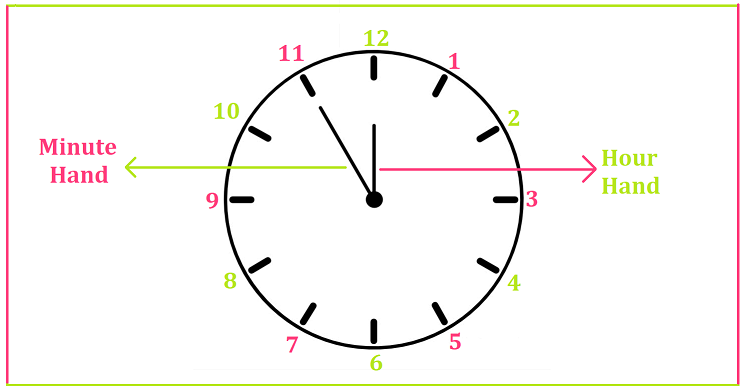The small hand shows hours.

The large hand shows minutes.

Half hour

There are 60 minutes in an hour.

30 minutes make half an hour. It is read as ‘half past the hour.'

At half past, the hour hand is halfway from one number to the next number.

For example,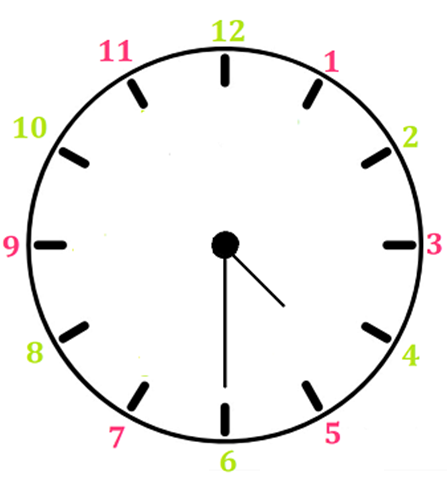In this clock, the hour hand is exactly between 4 and 5.

The minute hand points to 6 i.e., 30 minutes.

The time shown by the clock is half past 4.

Quarter Hour

Quarter means one-fourth.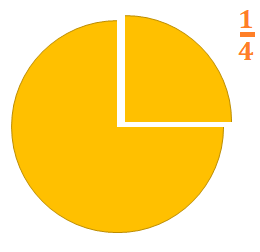An hour has 60 minutes.

A quarter of an hour means 15 minutes.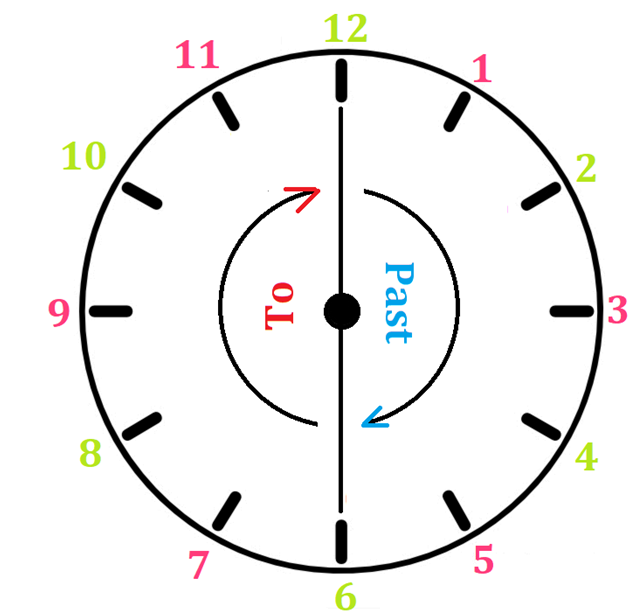When the minute hand points to 3, it is called ‘quarter past.’

When the minute hand points to 9, it is called ‘quarter to.’

Example 1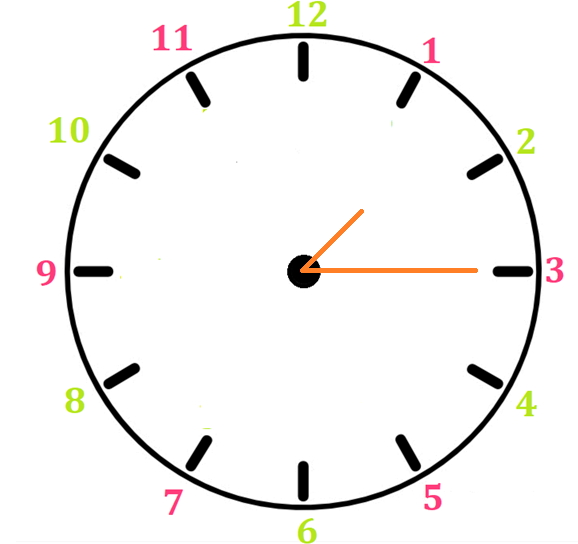The minute hand points to 3 and the hour hand is past 1.

The time shown by the clock is quarter past 1.

Example 2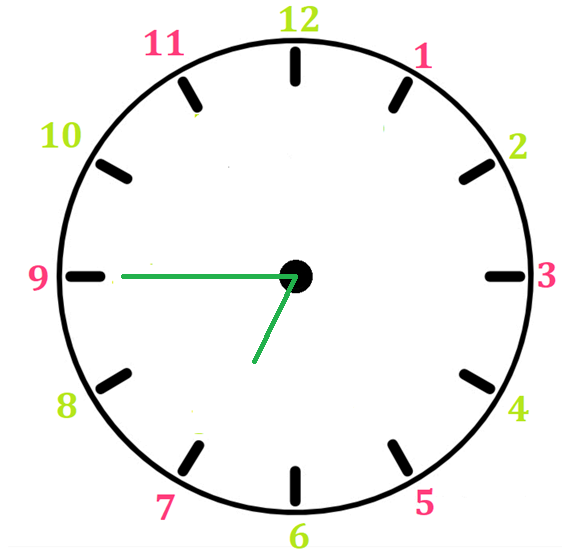The minute hand points to 9 and the hour is about to reach 7.

The time shown by the clock is quarter to 1.

//